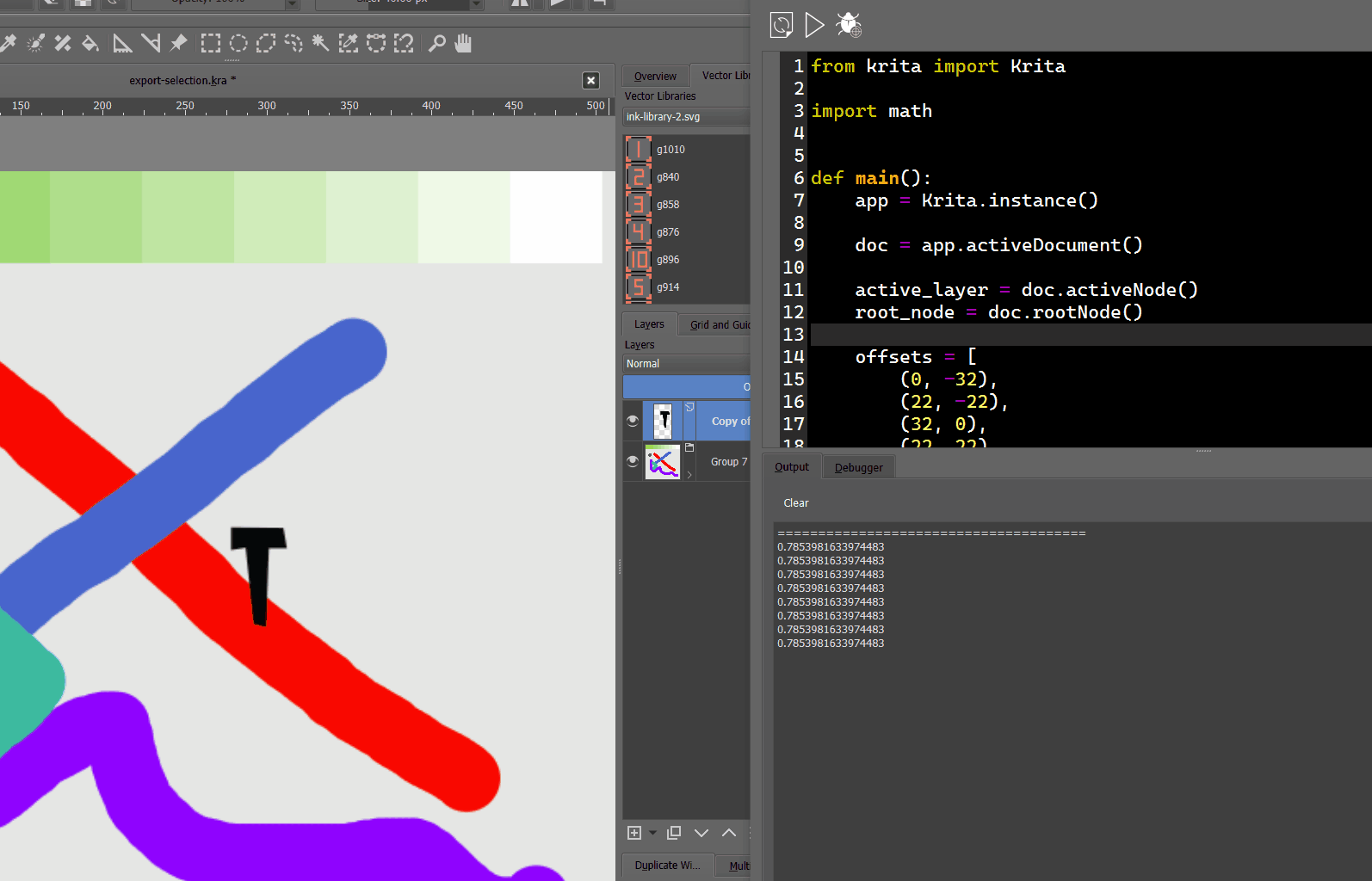# Node.move() is acting up

``````    active_layer = doc.activeNode()
bounds = active_layer.bounds()
center = bounds.center()
pos = active_layer.position()
x, y, w, h = bounds.x(), bounds.y(), bounds.width(), bounds.height()
print('center', type(center), center)
print('bounds', x, y, w, h)
print('position', pos)
# move x by width
active_layer.move(1, 1)
``````

I try to move the layer with `move()` method but it moves the thing outside the canvas.
Output for prints:

``````center <class 'PyQt5.QtCore.QPoint'> PyQt5.QtCore.QPoint(135, 335)
bounds 90 271 92 130
position PyQt5.QtCore.QPoint(494, 556)
``````

Is there something I am doing wrong?

Krita 4.4.8

Yes. If I apply move(34, 0) then the item is being thrown out of the canvas (on the left).

Any ideas why it happening and how to address this?

I was able to make smaller version of the script that acting up:

``````import re
import inspect

from pprint import pprint

from PyQt5.QtWidgets import QInputDialog, QDialog, QHBoxLayout, QVBoxLayout, QPushButton, QDialogButtonBox, QLabel, QSpinBox
from krita import Krita, Selection, InfoObject, Extension
from PyQt5.QtCore import QPoint

import math

def main():
app = Krita.instance()

doc = app.activeDocument()

active_layer = doc.activeNode()
root_node = doc.rootNode()

offsets = [
(0, -32),
(22, -22),
(32, 0),
(22, 22),
(0, 32),
(-22, 22),
(-32, 0),
(-22, -22)
]

iters = 8
for i, (x, y) in zip(range(iters), offsets):
angle = math.pi * 2 / iters
print(angle)

leaf = active_layer.duplicate()
leaf.rotateNode(i * angle)
leaf.move(x, y)
doc.waitForDone()
doc.refreshProjection()
``````

The common theme I noticed that `move` is acting out only for offsets that are vertical or horizontal.

Submitted a bug: 443361 – Node.move() after rotation is acting out

First of all, your example does not work since you aren’t calling main()

Second of all, move is not translate, it moves to the absolute position. So you need to subtract/add to the current position to translate.

Edit: Also another thing important to note after a rotation. When you rotate something that is slim to say diagonally, its bounding box will increase. So the position of that object may change depending how big the bounding box becomes.

Lastly, if you plan to do transformations like that, it may make more sense to load up the layer as pixdata or full sized thumbnail in a QImage and do a QTransform on the QImage, then output the results. This would save a lot of redrawing on the canvas and maybe some race conditions as well.

Hm. I thought main is being called automatically in scripter - at least what is done in my case.

That is weird. Because again by running experiments it looks like relative translate to meEven if the position would be absolute. Then for horizontal object (I highlight it with transform) the position should be somewhere around top left corner but instead it is roughly on (-250, 200) which is way farther than 30 pixels.

Hm. I see. So it is better to work with actual pixels manually instead of using API. Got it. ThanksI will give it a shot.

Not for me, it might be the difference between operating systems.

The position is based on the original position. For example, if you run:

``````doc = Krita.instance().activeDocument()
node = doc.activeNode()
node.move(10,10)
doc.refreshProjection()
print ( node.position() )
``````

multiple times, it only moves the 1st time, but not after. So it shouldn’t be confused with a translate which adds/subtracts based on current position.

It still confusing… I guess having this method called `setPostion` would make more sense.
`move` method has a lot of interpretations - and its behavior is way to unpredictable.

Thanks for looking at it @KnowZero , I will go with transformation on pixel data directly. At least there should be some consistencyVery Informative Conversation. Thanks Krita